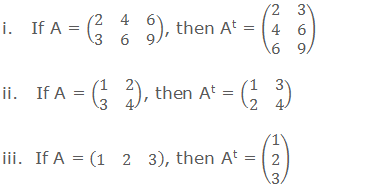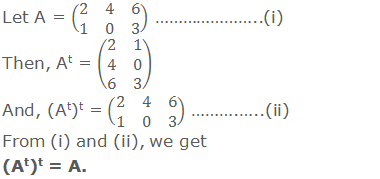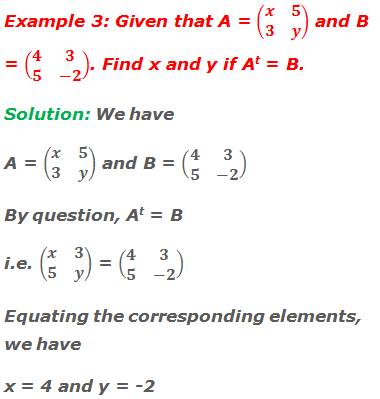# Transpose of a Matrix

## Transpose of a Matrix

Let A be a matrix. Then a new matrix obtained by interchanging the corresponding rows and columns of A is called the transpose of A. It is denoted by A’ or At.

********************

10 Math Problems officially announces the release of Quick Math Solver, an Android App on the Google Play Store for students around the world.

********************Here, the order of matrix A is 2 × 3 and that of At is 3 × 2. Hence if the order of a matrix A is m × n, then the order of transpose of matrix A i.e. At will be n × m. If A is a square matrix of order n, then its transpose At is also a square matrix of order n. If A is a row matrix, then its transpose At is a column matrix.For example:### Properties of Transpose of a Matrix

1.   The transpose of the transpose of a matrix is the matrix itself, i.e. (At)t = A.2.   The transpose of the sum of two matrices is equal to the sum of their transposes, i.e. (A + B)t = At + Bt.3.   If A is any matrix and k is any number, then (kA)t = kAt.4.   If A and B are two matrices conformable for multiplication, then (AB)t = BtAt.### Worked Out Examples#### Do you have any questions regarding the transpose of a matrix?

You can ask your questions or problems here, in the comment section below.